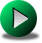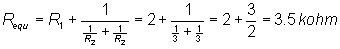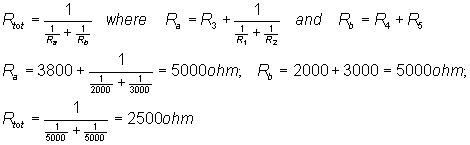# SERIES-PARALLEL CONNECTED RESISTORSClick or Tap the Example circuits below to invoke TINACloud and select the Interactive DC mode to Analyze them Online.
Get a low cost access to TINACloud to edit the examples or create your own circuitsPARALLEL CONNECTED RESISTORSWYE to DELTA and DELTA to WYE CONVERSION

In many circuits, resistors are connected in series in some places and in parallel in other places. To calculate the total resistance, you must learn how to distinguish between the resistors that are connected in series and the resistors that are connected in parallel. You should use the following rules:

1. Anywhere there is one resistor through which all the current flows, that resistor is connected in series.

2. If the total current is divided among two or more resistors whose voltage is the same, those resistors are connected in parallel.

Although we do not illustrate the technique here, you will often find it helpful to redraw the circuit so as to more clearly reveal the series and parallel connections. From the new drawing, you will be able to see more clearly how resistors are connected.

Example 1

Click/tap the circuit above to analyze on-line or click this link to Save under Windows

What is the equivalent resistance measured by the meter?

{Solution by TINA's Interpreter!}

Req:=R1+Replus(R2,R2);

Req=[3.5k]

You can see that the total current flows through R1, so it is series connected. Next, the current branches as it flows through two resistors, each labeled R2. These two resistors are in parallel. So the equivalent resistance is the sum of R1 and the parallel Req’ of the two resistors R2:The figure shows TINA's DC analysis solution.

Example 2

Click/tap the circuit above to analyze on-line or click this link to Save under Windows

Find the equivalent resistance measured by the meter.

Start at the "innermost" portion of the circuit, and note that R1 and R2 are in parallel. Next, note that R12=Req of R1 and R2 are in series with R3. Finally, R4 and R5 are series connected, and their Req is in parallel with the Req of R3, R1, and R2. This example shows that sometimes it is easier to start from the side furthest from the measuring instrument.{Solution by TINA' Interpreter!}

R12:=Replus(R1,R2)

Req:=Replus((R4+R5),(R3+R12));

Req=[2.5k]

Example 3

Find the equivalent resistance measured by the meter.

Click/tap the circuit above to analyze on-line or click this link to Save under Windows

Study the expression in the Interpreter box carefully, beginning inside the innermost parentheses. Again, as in Example 2, this is furthest from the ohmmeter. R1 and R1 are in parallel, their equivalent resistance is in series with R5, and the resultant parallel equivalent resistance of R1, R1, R5, and R6 is in series with R3 and R4, all of which is in parallel finally with R2.

{Solution by TINA's Interpreter!}

R1p:=Replus(R1,R1);

R6p:=Replus((R1p+R5),R6);

Req:=Replus(R2,(R3+R4+R6p));

Req=

Example 4

Find the equivalent resistance looking into the two terminals of this network.

Click/tap the circuit above to analyze on-line or click this link to Save under Windows

In this example, we have used a special 'function' of TINA's Interpreter called 'Replus' which calculates the parallel equivalent of two resistors. As you can see, using parentheses, you can calculate the parallel equivalent of more complicated circuits.

Studying the expression for Req, you can again see the technique of starting far from the ohmmeter and working from the "inside out."

{Solution by TINA's Interpreter!}

Req:=R1+R2+Replus(R3,(R4+R5+Replus(R1,R4)));

Req=

The following is an example of the well known ladder network. These are very important in filter theory, where some components are capacitors and/or inductors.

Example 5

Find the equivalent resistance of this network

Click/tap the circuit above to analyze on-line or click this link to Save under Windows

Studying the expression for Req, you can again see the technique of starting far from the ohmmeter and working from the "inside out."

First R4 is in parallel with the series connected R4 and R4.

Then this equivalent is in series with R and this Req is in parallel with R3.

This equivalent is in series a further R and this equivalent is in parallel with R2.

Finally this last equivalent is in series with R1 and their equivalent in parallel with R, wich equivalent is Rtot .

{Solution by TINA' Interpreter!}

{the network is a so called ladder}

R44:=Replus(R4,(R4+R4));

R34:=Replus(R3,(R+R44));

R24:=Replus(R2,(R+R34));

Req1:=Replus(R,(R1+R24));

Req1=[7.5]

{or in one step}

Req:=Replus(R,(R1+Replus(R2,(R+Replus(R3,(R+Replus(R4,(R4+R4))))))));

Req=[7.5]PARALLEL CONNECTED RESISTORSWYE to DELTA and DELTA to WYE CONVERSION